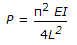# Civil Engineering - Theory of Structures - Discussion

### Discussion :: Theory of Structures - Section 2 (Q.No.35)

35.is the equation of Euler's crippling load, if

 [A]. both the ends are fixed [B]. both the ends are hinged [C]. one end is fixed and other end is free [D]. one end is fixed and other end is hinged.

Explanation:

No answer description available for this question.

 Vardhan said: (Aug 15, 2017) Here, Both ends are fixed.

 Asay said: (Nov 20, 2017) Euler,s Crippling Load Eqn is; P=(π)^2.EI/l^2. If, one end is fixed and other end is free Then , l=2L, Put it into above equation. P=(π)^2.EI/(2L)^2. P=(π)^2.EI/4L^4. So , C is the correct answer.

 Vidya said: (Feb 26, 2019) Both ends fixed, Le = L\2. Both ends hinged, Le = L. One end fixed one end free, Le = 2L. One end fixed one end hinged Le =L\√2.